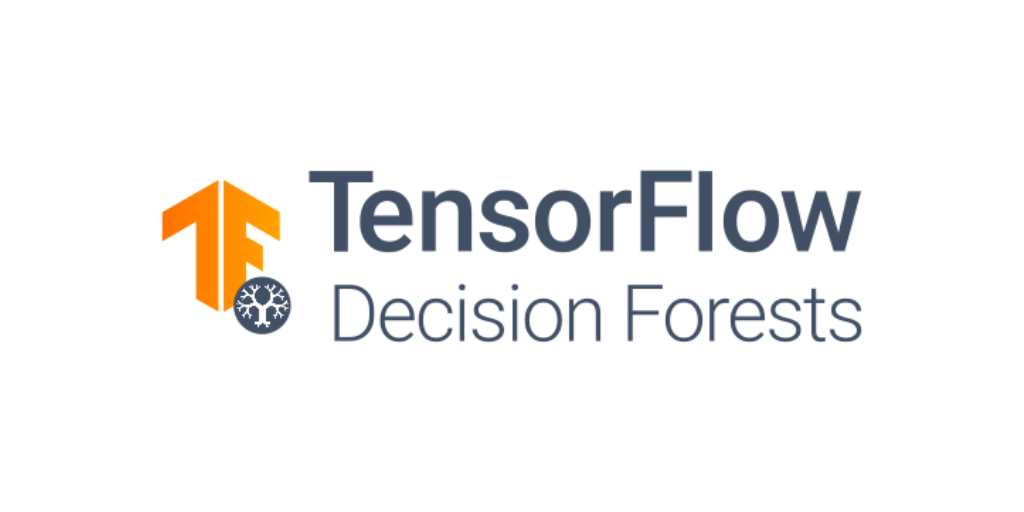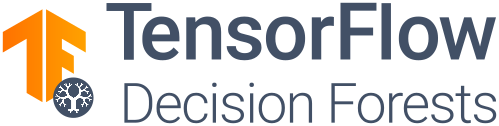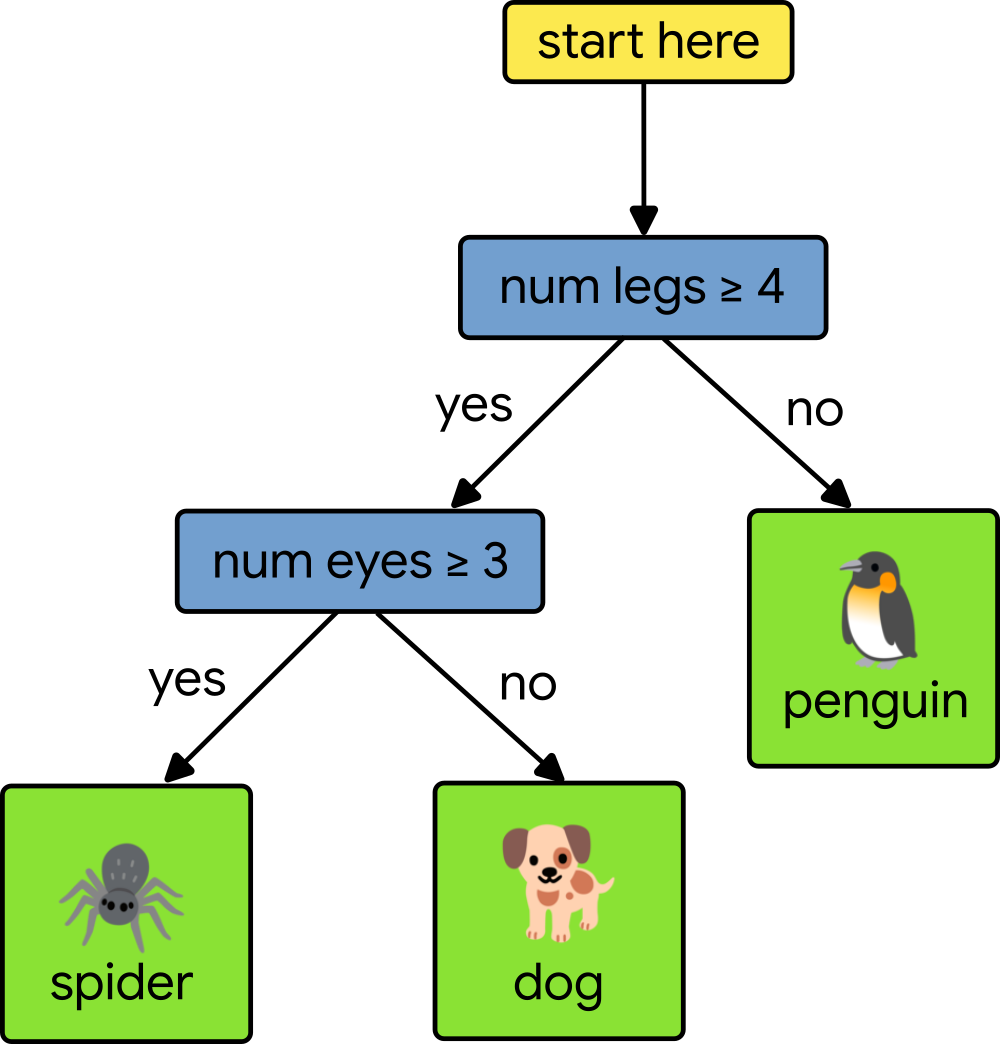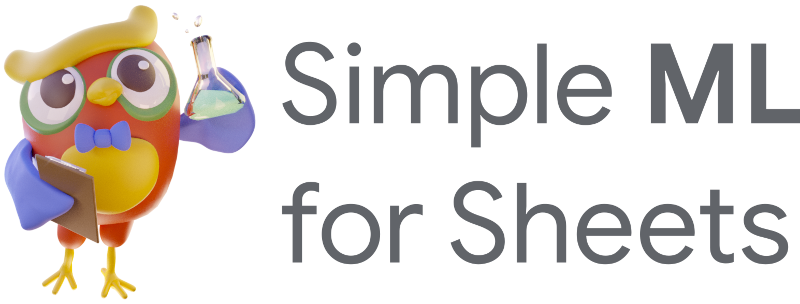CART · Decision Forests ·fevereiro 14, 2023Posted by Mathieu Guillame-Bert, Richard Stotz, Luiz GUStavo Martins

Two years ago, we open sourced the experimental version of TensorFlow Decision Forests and Yggdrasil Decision Forests, a pair of libraries to train and use decision forest models such as Random Forests and Gradient Boosted Trees in TensorFlow. Since then, we've added a lot of new features and improvements.Today, we are happy to announce that TensorFlow Decision Forests is production ready. In this post, we are going to show you all the new features that come with it 🙂. Buckle up!

### First, what are decision forests?

Decision forests are a type of machine learning model that train fast and work extremely well on tabular datasets. Informally, a decision forest is composed of many small decision trees. Together, they make better predictions thanks to the wisdom of the crowd principle. If you want to learn more, check out our class.If you're new to TensorFlow Decision Forests, we recommend that you try the beginner tutorial. Here is how easy it is to use TF-DF:

 ```train_df = pd.read_csv("train.csv") train_ds = tfdf.keras.pd_dataframe_to_tf_dataset(train_df, label="species") model = tfdf.keras.GradientBoostedTreesModel() model.fit(train_ds) model.save("my_model")```

Following are the main new features introduced to TensorFlow Decision Forests (TF-DF) in the 1.x release.

### Easier hyper-parameter tuningLike all machine learning algorithms, Decision Forests have hyper-parameters. The default values of those parameters give good results, but, if you really want the best possible results for your model, you need to "tune" those parameters.

TF-DF makes it easy to tune parameters. For example, the objective function and the configuration for distribution are selected automatically, and you specify the hyper-parameters you wish to tune as follows:

 ```tuner = tfdf.tuner.RandomSearch(num_trials=50) tuner.choice("min_examples", [2, 5, 7, 10]) tuner.choice("categorical_algorithm", ["CART", "RANDOM"]) tuner.choice("max_depth", [3, 4, 5, 6, 8]) tuner.choice("use_hessian_gain", [True, False]) tuner.choice("shrinkage", [0.02, 0.05, 0.10, 0.15]) tuner.choice("growing_strategy", ["LOCAL"]).choice("max_depth", [3, 4, 5, 6, 8]) tuner.choice("growing_strategy", ["BEST_FIRST_GLOBAL"], merge=True).choice("max_num_nodes", [16, 32, 64, 128, 256]) # ... Add all the parameters to tune model = tfdf.keras.GradientBoostedTreesModel(verbose=2, tuner=tuner) model.fit(training_dataset```

Starting with TF-DF 1.0, you can use the pre-configured hyper-parameter tuning search space. Simply add `use_predefined_hps=True` to your model constructor and the tuning will be done automatically:

 ```tuner = tfdf.tuner.RandomSearch(num_trials=50, use_predefined_hps=True) # No need to configure each hyper-parameters tuned_model = tfdf.keras.GradientBoostedTreesModel(verbose=2, tuner=tuner) tuned_model.fit(train_ds, verbose=2) ```

Check the hyper-parameter tuning tutorial for more details. And, if your dataset is large, or if you have a lot of parameters to optimize, you can even use distributed training to tune your hyper-parameters.

### Hyper-parameters templates

As mentioned above, to maximize the quality of your model you need to tune the hyper-parameters. However, this operation takes time. If you don't have the time to tune your hyper-parameters, we have a new solution for you: Hyper-parameter templates.

Hyper-parameter templates are a set of hyper-parameters that have been discovered by testing hundreds of datasets. To use them, you simply need to set the `hyperparameter_template` argument.

 ```model = tfdf.keras.GradientBoostedTreesModel(hyperparameter_template="benchmark_rank1") model.fit(training_dataset) ```

In our paper called "Yggdrasil Decision Forests: A Fast and Extensible Decision Forests Library", we show experimentally that the results are almost as good as with manual hyper-parameter tuning.

See the "hyper-parameter templates" sections in the hyper-parameter index for more details.

### Serving models on Google CloudTensorFlow Decision Forests is now included in the official release of TensorFlow Serving and in Google Cloud's Vertex AI. Without any special configuration or custom images, you can now run TensorFlow Decision Forests in Google Cloud.

See our examples for TensorFlow Serving.

### Distributed training on billions of examplesTraining TF-DF on datasets with less than a million examples is almost instantaneous. On larger datasets however, training takes longer. TF-DF now supports distributed training. If your dataset contains multiple millions or even billions of examples, you can use distributed training on tens or even hundreds of machines.

Here is an example:

 ```cluster_resolver = tf.distribute.cluster_resolver.TFConfigClusterResolver() strategy = tf.distribute.experimental.ParameterServerStrategy(cluster_resolver) with strategy.scope(): model = tfdf.keras.DistributedGradientBoostedTreesModel( temp_directory=..., num_threads=30, ) model.fit_on_dataset_path( train_path=os.path.join(dataset_path, "train@60"), valid_path=os.path.join(dataset_path, "valid@20"), label_key="my_label", dataset_format="csv") ```

See our end-to-end example and documentation for more details and examples.

### Training models in Google Sheets

To make it even easier to train decision forests, we created Simple ML for Sheets. Simple ML for Sheets makes it possible to train, evaluate, and interpret TensorFlow Decision Forests models in Google Sheets without any coding!And once you have trained your model in Google Sheets, you can export it back to TensorFlow Decision Forests and use it like any other models.

Check the Simple ML for Sheets tutorial for more details.

### Next steps

We hope you enjoyed reading this news, and that the new version of TensorFlow Decision Forests will be useful for your work.